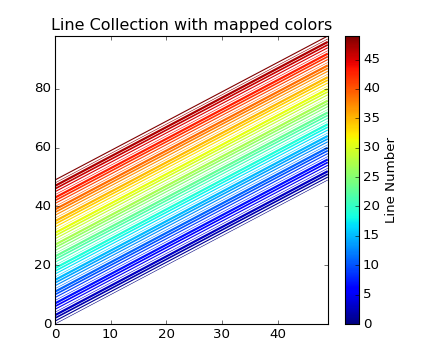### We're updating the default styles for Matplotlib 2.0

Learn what to expect in the new updates#### Previous topic

pylab_examples example code: line_collection.py

# pylab_examples example code: line_collection2.py¶```from pylab import *
from matplotlib.collections import LineCollection

# In order to efficiently plot many lines in a single set of axes,
# Matplotlib has the ability to add the lines all at once. Here is a
# simple example showing how it is done.

N = 50
x = arange(N)
# Here are many sets of y to plot vs x
ys = [x+i for i in x]

# We need to set the plot limits, they will not autoscale
ax = axes()
ax.set_xlim((amin(x),amax(x)))
ax.set_ylim((amin(amin(ys)),amax(amax(ys))))

# colors is sequence of rgba tuples
# linestyle is a string or dash tuple. Legal string values are
#          solid|dashed|dashdot|dotted.  The dash tuple is (offset, onoffseq)
#          where onoffseq is an even length tuple of on and off ink in points.
#          If linestyle is omitted, 'solid' is used
line_segments = LineCollection([list(zip(x,y)) for y in ys], # Make a sequence of x,y pairs
linewidths    = (0.5,1,1.5,2),
linestyles = 'solid')
line_segments.set_array(x)